Function Repository Resource:

# TsallisEntropy

Compute the Tsallis entropy

Contributed by: Julián Laverde and Mads Bahrami
 ResourceFunction["TsallisEntropy"][prob,q,k] returns the Tsallis entropy for a list of discrete associated probablities prob of the possible configurations for the entropic index q and constant k. ResourceFunction["TsallisEntropy"][dist,q,k] returns the Tsallis entropy of the probability distribution dist, entropic index q and constant k. ResourceFunction["TsallisEntropy"]["formula"] returns the specified formula of the Tsallis entropy.

## Details

Tsallis entropy is a generalization of the Boltzmann–Gibbs–Shannon entropy.
For a discrete set of probabilities {pi}, the Tsallis entropy is defined as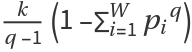.
Tsallis entropy Sq can equivalently be written as, or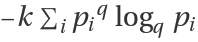, or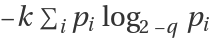, where the q-logarithm logqz is defined as logqz=(z1-q-1)/(1-q), with log1z= log z being the limiting value as q1.
For a continuous probability distribution p(x), the Tsallis entropy is defined as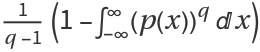.
ResourceFunction["TsallisEntropy"][prob,q,k] computes the Tsallis entropy for a given discrete set of probabilities prob={pi} with the condition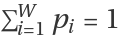, where W=Length[prob] is the number of possible configurations. If prob is not normalized, it will be normalized first.
In the limit as q1 for ResourceFunction["TsallisEntropy"][], the Shannon differential entropy is recovered.
In ResourceFunction["TsallisEntropy"][prob,q,k], prob can be a list of real numbers. q and k can be real numbers.
In ResourceFunction["TsallisEntropy"][dist,q,k], dist can be any built-in continuous or discrete distribution in the Wolfram Language, or a mixture of them.
If not given, the default value of k is 1.
In ResourceFunction["TsallisEntropy"]["formula"], "formula" can be any of the following:
 "DiscreteFormula" formula for Tsallis entropy for a discrete set of probabilities "ContinuousFormula" formula for Tsallis entropy for a continuous probability distribution

## Examples

### Basic Examples (3)

Compute the Tsallis entropy for a discrete set of probabilities from a list:

 In:=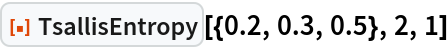Out=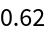The Tsallis entropy for a built-in discrete probability distribution:

 In:=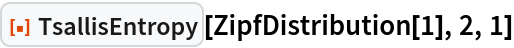Out=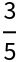The Tsallis entropy for a continuous probability distribution:

 In:=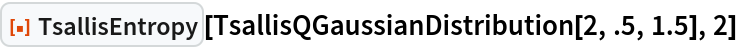Out=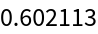### Scope (2)

The formula for the Tsallis entropy of a discrete set of probabilities:

 In:=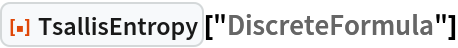Out=The formula for the Tsallis entropy of a continuous probability distribution:

 In:=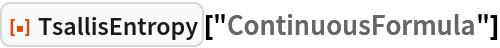Out=### Possible Issues (1)

In TsallisEntropy[prob,q,k], all elements of the list prob must be positive:

 In:=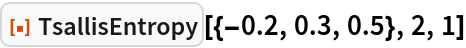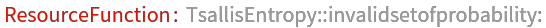Out=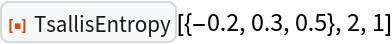### Neat Examples (4)

Tsallis entropy is concave for q>0, and convex for q<0. Plot Sq/k versus p:

 In:=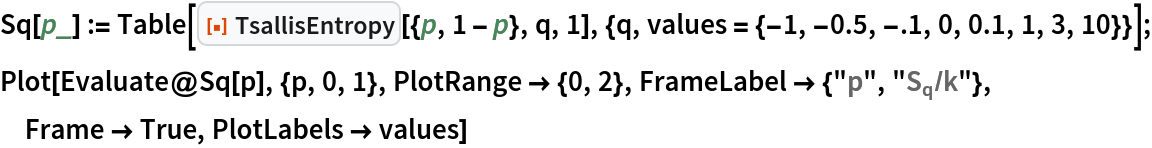Out=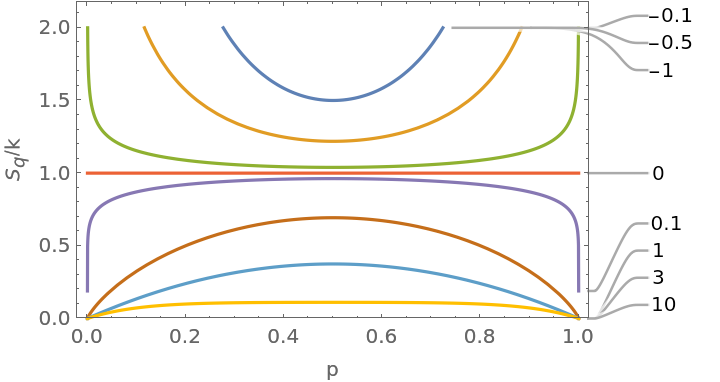Plot the value of the Tsallis entropy at its extremum (equiprobability) for k=1 and typical values of q:

 In:=Out=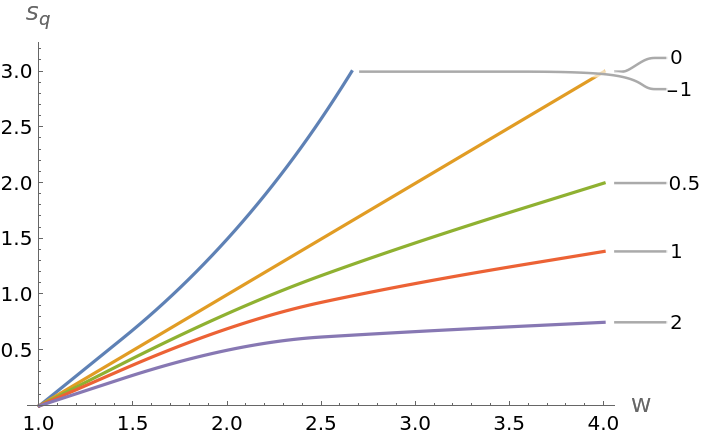Show non-additivity Sq(A,B)=Sq(A)+Sq(B)+(1-q)Sq(A)Sq(B) for two independent systems A and B with discrete sets of probability:

 In:=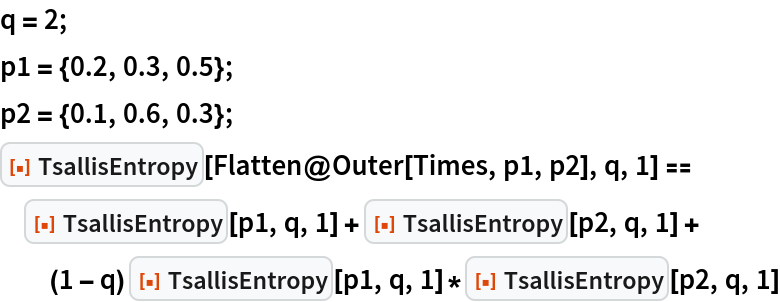Out=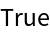Show non-additivity Sq(A,B)=Sq(A)+Sq(B) with q=1 for two independent systems A and B with continuous probability distributions:

 In:=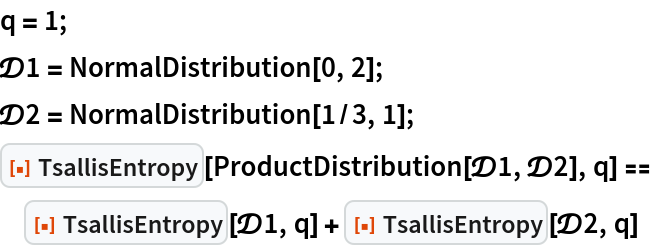Out=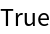## Publisher

WolframSpecialProjects

## Version History

• 1.1.0 – 12 April 2021
• 1.0.0 – 23 March 2021